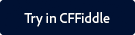Description

Determines the integer number of units by which date1 is less than date2.

Returns

A number of units, of type datepart.

Category

Date and time functions

Function syntax

`DateDiff(datepart, date1, date2)`

History

ColdFusion MX:

• Changed how negative date differences are calculated: this function calculates negative date differences correctly; its output may be different from that in earlier releases.

Parameters

Parameter

Description

datepart

String that specifies the units in which to count; for example yyyy requests a date difference in whole years.

• yyyy: Years
• q: Quarters
• m: Months
• y: Days of year (same as d)
• d: Days
• w: Weekdays
• ww: Weeks
• h: Hours
• n: Minutes
• s: Seconds

Note: The mask w as weekdays is only valid for the 2018 release of ColdFusion. For 2016 and earlier versions of ColdFusion, w returns the number of weeks.

date1

date2

Usage

The DateDiff function determines the number of complete datepart units between the two dates; for example, if the datepart parameter is "m" and the dates differ by 55 days, the function returns 1.Enclose string constant dates in quotation marks. If the text contains only numbers (such 1932), and is not surrounded by quotation marks, ColdFusion interprets it as a date/time object, resulting in an incorrect value.

Note:

In the DateDiff function, there is a difference in how the date difference is calculated for the member function. For example, in the code below,

```<cfscript>
```
```    date1="2018-09-25"
```
```    date2="2018-10-25"
```
```    diffA=dateDiff("ww", date1, date2)
```
```    writeOutput(diffA) // 4
```
```    diffB=date1.diff("ww",date2)
```
```    writeOutput(diffB) // -4
```
```</cfscript>
```The first writeOutput produces a difference of 4 weeks, whereas the second writeOutput produces a difference of -4 weeks. Using DateDiff, the difference is calculated as date2-date1, whereas using the member function, the difference is calculated as date1-date2.

It is a known behavior in ColdFusion and the results are as expected.

Example

```<cfscript>
Date1 = "{ts '2018-11-15 12:13:50'}";
Date2 = "{ts '2018-12-15 12:13:50'}";
diff= DateDiff("d",Date1,Date2)
diff1= DateDiff("ww",Date1,Date2)
diff2= DateDiff("m",Date1,Date2)
writeOutput("difference in date is : " & diff &  " days or" & "<br/>" )
writeOutput("difference in date is : " & diff1 & " weeks or" & "<br/>")
writeOutput("difference in date is : " & diff2 & " month" & "<br/>")
</cfscript>```

Output

difference in date is : 30 days or
difference in date is : 4 weeks or
difference in date is : 1 monthTwitter™ and Facebook posts are not covered under the terms of Creative Commons.